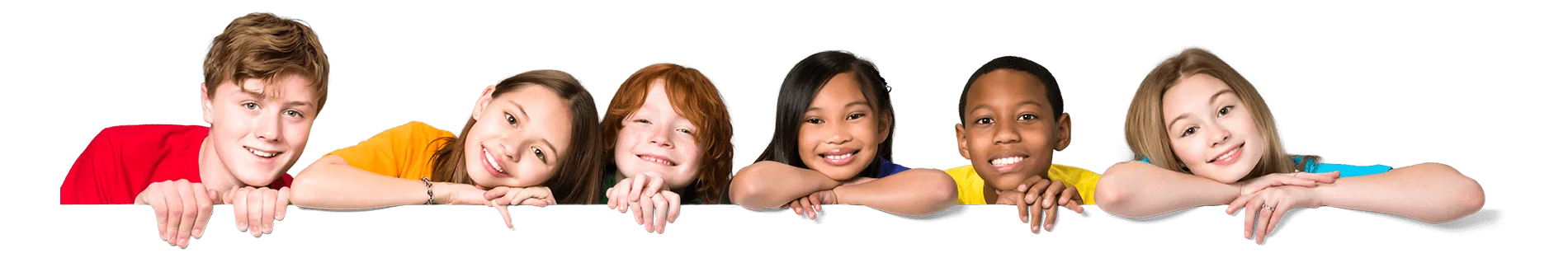Latest Results:

# 11 Plus 2D and 3D Shapes

Geometry is the most interesting, easy and most used part of mathematics. It may be any field we will be using geometry like construction, machine-building, even rocket sciences use it. 2D and 3D shapes are considered to be the most important parts of geometry. Here D indicates dimension. The 2D shape is a 2 Dimensional shape, which lies on a plane (an infinite imaginary sheet). If we add width to the 2D, we get 3D shapes.  Some examples of 2D shapes are Triangle, Square, Circle etc. if we add something like width or height to them, they turn to 3D. If we add height to the square, it turns to Cube; similarly, if we add height to circle, it turns to Cylinder. Some other 3D shapes are cone, pyramid, cuboid, etc.. 2D and 3D shapes are 11 plus geometry.

Considering 2D shapes initially, we will learn many interesting things about these shapes right from the basics.

Let’s have a glance at some of the shapes,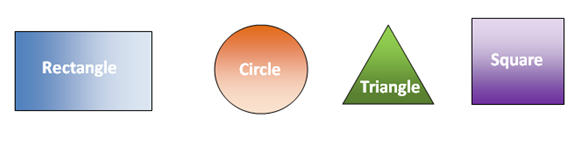The above-shown shapes are some examples of 2d shapes. If we observe them clearly, except the circle remaining are made of line segments (the line segment is a part of the line with two endpoints), the circle is not made of line segments but made of arcs.

Do you know you can draw any 2D shape by tracing out the object you have, like a book, food cans?

Exercise: Observe the figure and try to find out the shapes from it.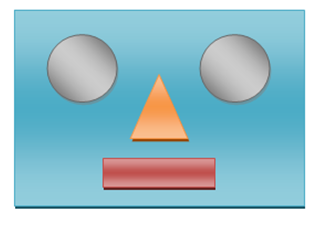From the above figure, we can find 2 circles (eyes), a triangle (nose), and two rectangles (face and mouth). So it is easy to make some funny faces with these basic shapes, so what are you waiting for? Take a paper and try to draw some pictures using 2D shapes.

Let’s know about them and their properties in detail simply and effectively, starting with a circle.

Circles: A circle is a 2D shape in which all points lying on the edge of the circle are at the same distance from the centre—the important parameters of circle diameter, radius, chord, centre, etc.In the above figure point, O is known as the centre, AB is known as Diameter, PQ is known as Chord and OR is known as the circle's radius.

• A line segment connecting any two points on the circle is known as a chord. The chord passing through the centre of the circle is known as diameter.
• The diameter is said to be the longest chord of the circle.
• We can have an infinite number of Diameters in a circle.
• Every diameter is a chord, but every chord need not be the diameter.
• The diameter divides the circle into two halves, and each half is known as Semicircle.
• A line segment connecting the centre and any point on the circle is known as the circle's radius.
• Every circle has an infinite number of Radii.
• If the radius is 0, then it is called a Point Circle.
• If the radius is one, then it is called a Unit Circle.
• Two circles with a common centre and different radii are said to be Concentric Circles.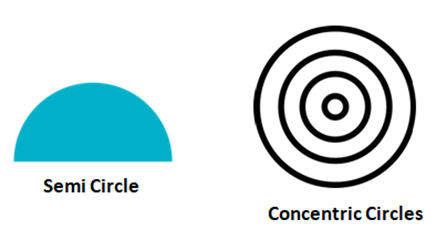The circle area is considered the space occupied by the circle, and the perimeter is considered the boundary of the circle and sometimes called the length of the circle.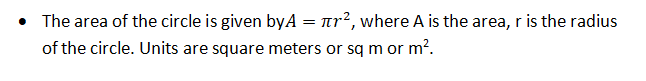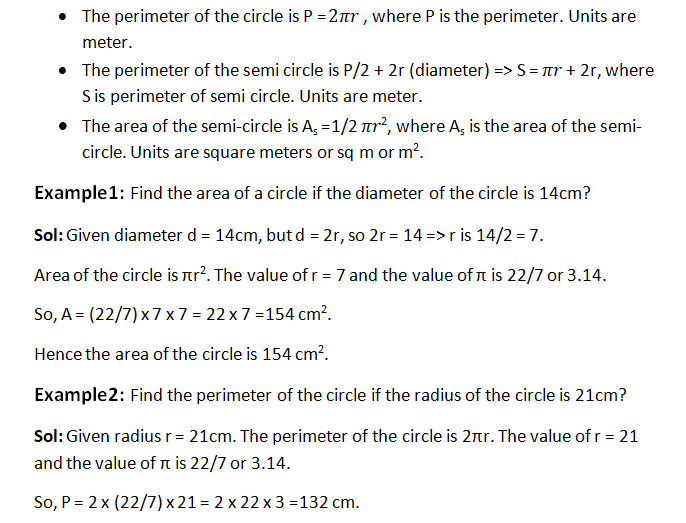Hence perimeter of the circle is 132 cm.

The other shapes made of a Line segment are called Polygons. Polygons are defined as the closed shapes made of line segments. Based on the number of line segments used, polygons have names. Suppose three-sided polygons are known as Triangles, four-sided one is called Quadrilateral etc.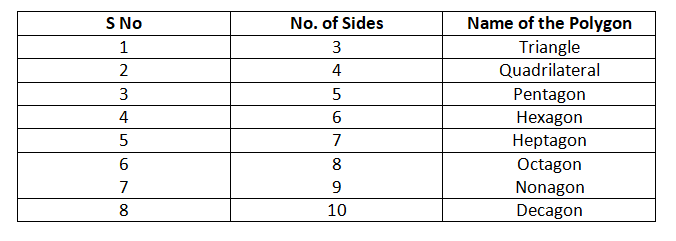Note: The perimeter of any polygon is just the sum of all the sides of that polygon. For example, a, b and c are the sides of the triangle, and its perimeter is a+b+c

The polygons Triangle and Quadrilateral are mostly used, so let’s learn about them in detail.

Triangle: Triangle is a three-sided polygon the name TRI ANGLE itself indicates it is a three-sided shape, with three angles (rotation made by any of 2 adjacent sides). They can be classified into many types based on sides and angles.

Types of Triangles

Based on the sides:

1. Equilateral Triangle
2. Isosceles Triangle
3. Scalene Triangle

Based on Angles:

1. Right-angled triangle
2. Obtuse angled triangle
3. Acute angled triangle
• The triangle area is given by (1/2) x bxh where b = base, h = height.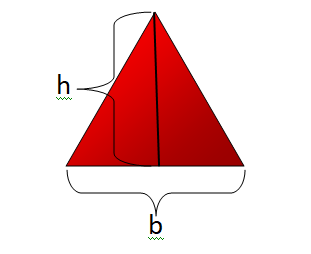Quadrilaterals: Four-sided polygon is called a Quadrilateral. Quad means 4. Some of the examples of quadrilaterals are square, rectangle, rhombus, trapezium, parallelogram etc...

• The area of a square of side ‘a’ is A = axa.
• Area of Rectangle of length l, breadth b is A = lxb.
• The area of Rhombus of diagonals p and q is A = (pxq) /2.
• Area of Parallelogram of side b and height h is A = bxh.
• Area of Trapezium of parallel sides a, b and height h is A = (a+b)/2 x h.

3D shapes:

They are 3-dimensional shapes that have width extra. The 3D shape of the square is Cube, the circle is Cylinder, and the rectangle is Cuboid.

Let’s see some examples of 3D shapes.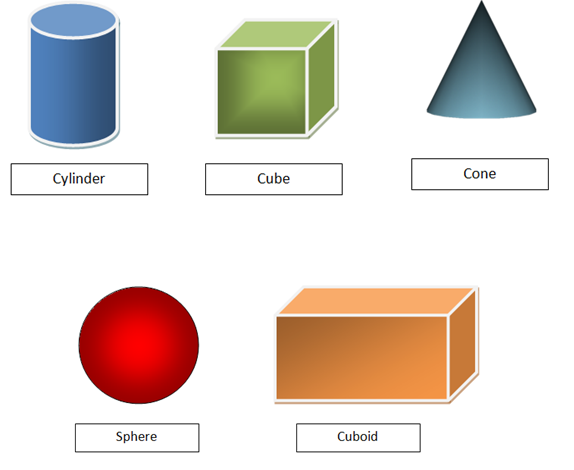Examples: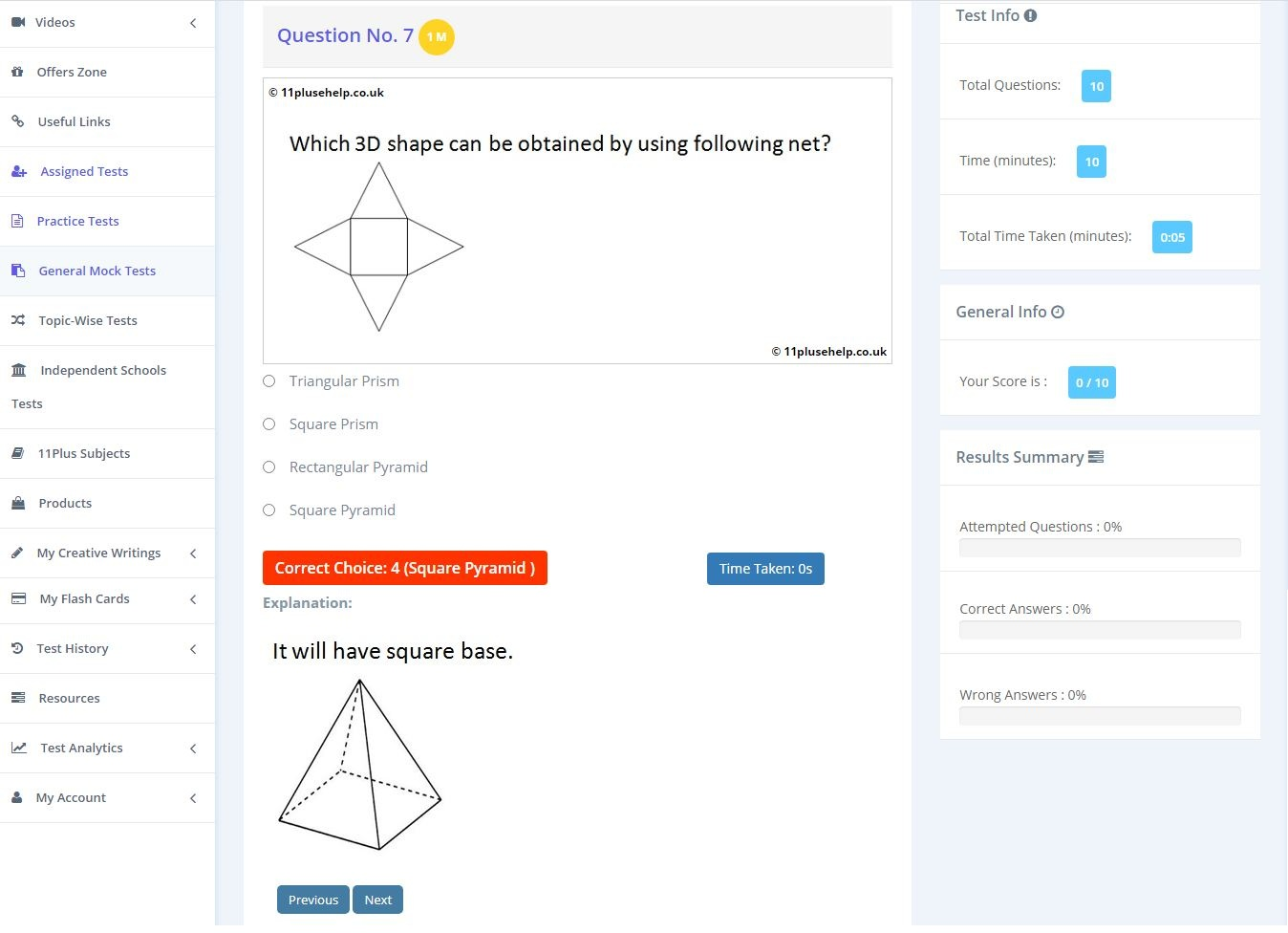You can access 11 plus practice papers on different topics here:

https://www.11plusehelp.co.uk/11-plus-maths-practice-tests

11PluseHelp.co.uk is a one-stop shop for 11 Plus exam preparation, including 11 Plus Independent schools preparation

There are many 11 Plus practice papers for entrance exams, Independent school papers and more for the 11 tests. Ranging from 10 to 25 minutes, our multiple-choice and fill-up question types are ideal for Maths revision.

At 11plusehelp.co.uk/, we possess the following resources:

Maths- 11 Plus Maths Worksheets, 11 Plus Maths Papers free download, 11 Plus Maths topics pdfs.

English- 11 Plus English papers with answers, 11 Plus comprehension with answers, 11 English comprehensions multiple-choice, English reasoning test.

Verbal and Non-Verbal Reasoning- 11 Plus Verbal Reasoning worksheets free, Verbal and Non-Verbal Reasoning tests, easy Non-Verbal Reasoning Worksheets

Practice Tests- Eleven plus sample papers, free 11 plus papers with answers, 11 plus mock test papers pdf, 11 exam question and answers, UK 11 plus exam questions, 11 online tests in Maths and English with 11 Plus answers.

We also have 11 plus resources for parents with 11 plus guide.

Our Independent School resources include-

Grammar school test papers with answers

KENT- KENT test practice papers, KENT 11 Plus

Bexley - Bexley 11 Plus Test, Bexley 11 Plus Solved Papers, Bexley 11 Plus Comprehension with Answers

KS2- Maths Reasoning KS2, Online Math Test KS2

These tests are useful for preparing 11+ CEM, CSSE and GL assessment and any other 11 Plus entrance examinations in the UK.

CEM- CEM assessments, CEM 11 papers pdf, CEM 11 Familiarization papers:https://www.11plusehelp.co.uk/11-plus-exam-papers/11-plus-cem-exam

GL assessment - https://www.11plusehelp.co.uk/11-plus-gl-assessment-exam

Top 100 Independent School Details:https://www.11plusehelp.co.uk/top-100-independent-schools

All Grammar Schools Details:https://www.11plusehelp.co.uk/list-of-grammar-schools

Revision Pack and guide free advice. Pack your bags to the grammar school/ private school you dream of with all this preparation.

11 Plus Revision Pack:

https://www.11plusehelp.co.uk/11-plus-practice-papers/11-plus-english-practice-test-papers/revision-pack

We focus on fundamentals, logic, basics and cover most of the syllabus for all 11 Plus exam patterns in the UK. For example, We cover Maths topic wise questions and fundamentals and how to apply them. We have constantly been adding practice papers regularly.

We have loads of questions useful for preparing for CEM, CSSE and GL assessment and any other 11 Plus entrance exams in the UK. Children will love to take these tests, and learning is fun at 11plusehelp.co.uk.

To access Virtual Mock Exams, please visit:  https://11plus-mockexams.co.uk/

You can access 11 Plus FREE Papers by visiting the below link:
https://www.11plusehelp.co.uk/11-plus-free-online-papers

You can access 11 Plus FREE Sample Papers by visiting the below link:
https://www.11plusehelp.co.uk/11-plus-sample-papers

11 Plus complete solution features:

https://11plusehelp.co.uk/blog/2017/05/01/11-plus-complete-solution/

Practice and Perseverance Over Genius and Talent

Thanks,

11Plusehelp.co.uk